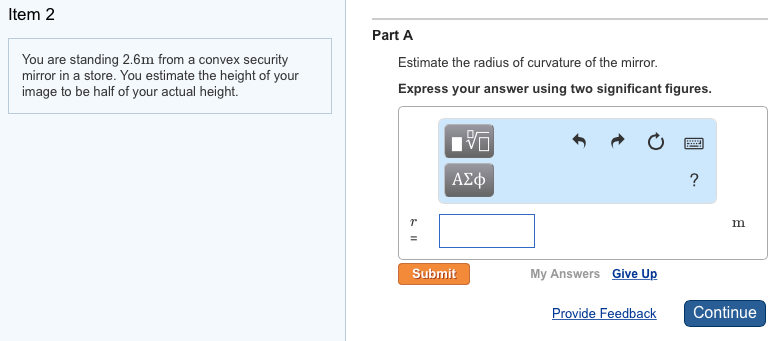Item 2 You are standing 2.6m from a convex security mirror in a store. You estimate the height of your image to be half of your actual height. Part A Estimate the radius of curvature of the mirror. Express your answer using two significant figures.

Object distance u = -2.6m
Let image distance = v
Height of image = half of height of object
so magnification = 0.5
Now magnification = -v/u
So -v/u = 0.5
v= -0.5u

Using mirror formula,
1/f=1/v + 1/u = 1/(-0.5u) + 1/u = -1/u
So f= -u = 2.6m
R=2f =2 x 2.6 = 5.2m

#### Earn Coin

Coins can be redeemed for fabulous gifts.

Similar Homework Help Questions
• ### You are standing 4.5m from a convex security mirror in a store. You estimate the height...

You are standing 4.5m from a convex security mirror in a store. You estimate the height of your image to be half of your actual height. Estimate the radius of curvature of the mirror. Follow the sign conventions.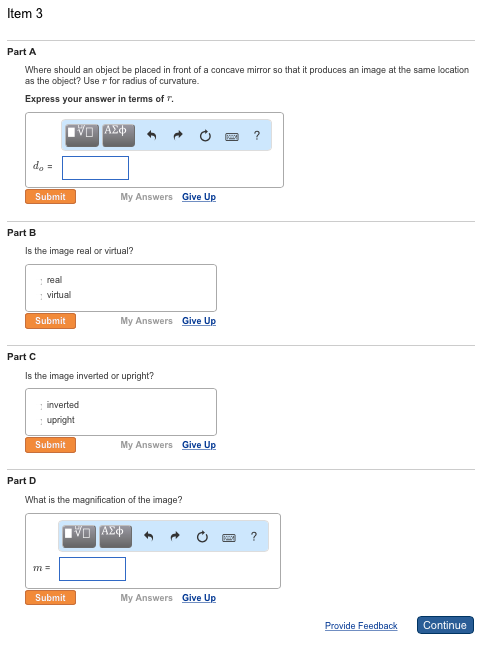11 : 3 : Please help me answer this question: Item 3 Part A Where should w object be placed in front of a concave mirror so that it produces en image at the swne location as the object? Use r for radius of curvature Express your answer in terms of r. d0 = Part B Is the image reel or virtual? real virtual Part C Is the image inverted or upright? inverted upright Part D Whet is the magnification...

• ### A guy named Joe, who is 1.6 meters tall, enters a room in which someone has...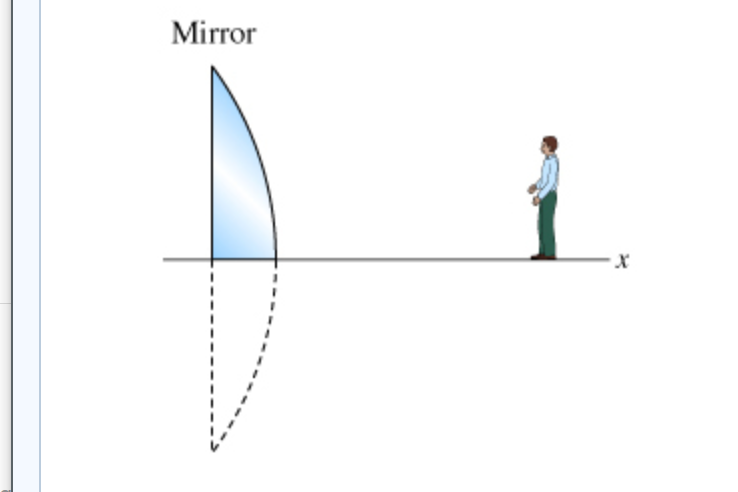A guy named Joe, who is 1.6 meters tall, enters a room in which someone has placed a large convex mirror with radius of curvature R equal to 30 meters. The mirror has been cut in half, so that the axis of the mirror is at ground level. (Figure 1) As Joe enters the room, he is 5 meters in front of the mirror, but he is looking the other way, so he fails to see it. When he turns...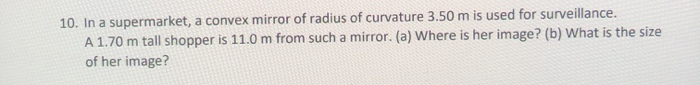please help me in this,thank you! 10. In a supermarket, a convex mirror of radius of curvature 3.50 m is used for surveillance. A 1.70 m tall shopper is 11.0 m from such a mirror. (a) Where is her image? (b) What is the size of her image?

• ### am needing help, thank you!! Review | Constants A 3.0 cm tall object is 55 cm...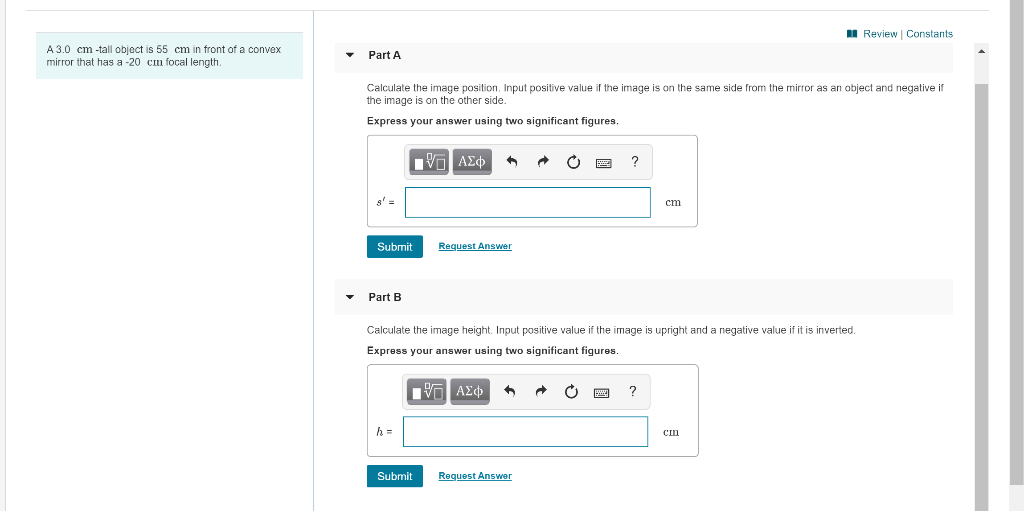am needing help, thank you!! Review | Constants A 3.0 cm tall object is 55 cm in front of a convex mirror that has a -20 cm focal length. Part A Calculate the image position. Input positive value if the image is on the same side from the mirror as an object and negative if the image is on the other side. Express your answer using two significant figures. nνα ΑΣφ cm Request Answer Submit Part B Calculate the image...

• ### can you please solve this Question 21 5 pts A concave mirror in an amusement park...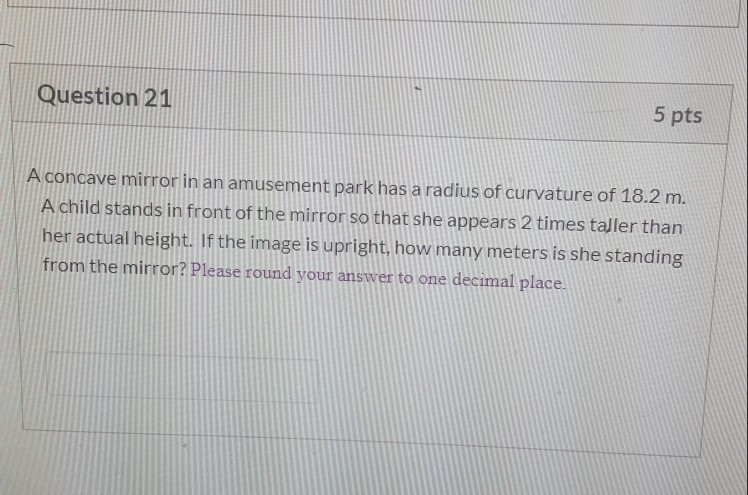can you please solve this Question 21 5 pts A concave mirror in an amusement park has a radius of curvature of 18.2 m. A child stands in front of the mirror so that she appears 2 times taller than her actual height. If the image is upright, how many meters is she standing from the mirror? Please round your answer to one decimal place.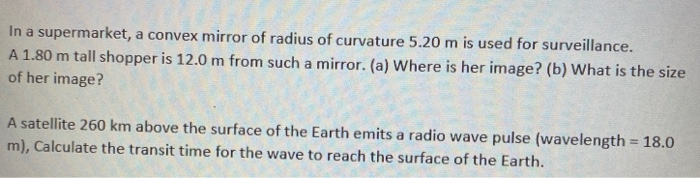please help me and thank you in advance In a supermarket, a convex mirror of radius of curvature 5.20 m is used for surveillance. A 1.80 m tall shopper is 12.0 m from such a mirror. (a) Where is her image? (b) What is the size of her image? A satellite 260 km above the surface of the Earth emits a radio wave pulse (wavelength = 18.0 m), Calculate the transit time for the wave to reach the surface of...

• ### Figure 1 Figure 2 Part A Consider the following diagrams noting that the scale is different between di...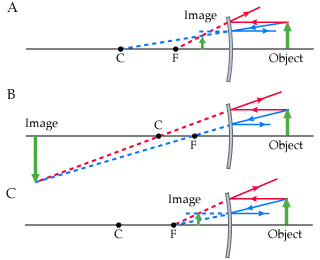Figure 1 Figure 2 Part A Consider the following diagrams noting that the scale is different between diagrams. In these diagrams, C and F represent the center of curvature and the focal point of the convex mirror, respectively. The image formed by the mirror is obtained using the ray tracing technique. Which diagram(s) are correct?(Figure 1) TypeAif you think that only diagram A is correct, typeABif you think that only diagrams A and B are correct, and so on. Part...

• ### An object is placed 30 cm from a certain mirror. The image is half the size...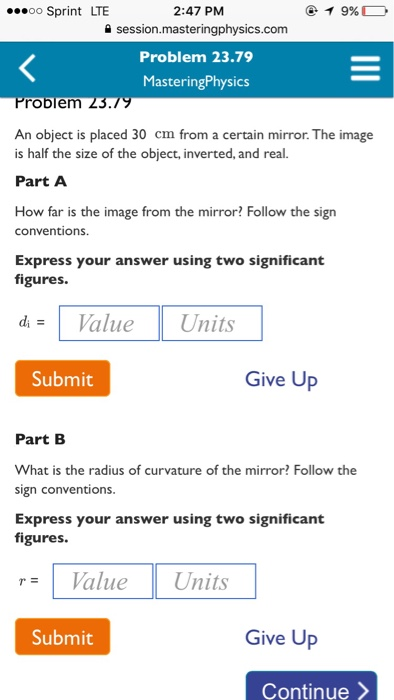An object is placed 30 cm from a certain mirror. The image is half the size of the object, inverted, and real. How far is the image from the mirror? Follow the sign conventions. Express your answer using two significant figures. What is the radius of curvature of the mirror? Follow the sign conventions. Express your answer using two significant figures.

• ### A ray diagram can be used to find the location of an object if you are...

A ray diagram can be used to find the location of an object if you are given the location of its image and the focal length of the mirror. Part A Draw a ray diagram to find the height and position of an object that makes a 1.7-cm-high upright virtual image that appears 8.0 cm behind a convex mirror of focal length 25 cm . Express your answer using two significant figures. p= Part B Express your answer using two...

Free Homework Help App